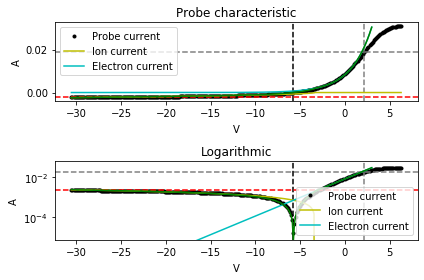Important

The Analysis and Diagnostic framework is in active development at the moment. For the foreseeable future, the API will be in continuous flux as functionality is added and modified. To follow the package development please visit our GitHub Project ( https://github.com/PlasmaPy/PlasmaPy/projects/19 ) and comment on any of the relevant issues and/or pull requests.

Warning

This module will be deprecated in favor of .

# Langmuir analysis

Defines the Langmuir analysis module as part of the diagnostics package.

## Classes

 Characteristic(bias, current) Class representing a single I-V probe characteristic for convenient experimental data access and computation.## Functions

 extract_exponential_section(probe_characteristic) Extract the section of exponential electron current growth from the probe characteristic. extract_ion_section(probe_characteristic) Extract the section dominated by ion collection from the probe characteristic. extrapolate_electron_current(...[, ...]) Extrapolate the electron current from the Maxwellian electron temperature obtained in the exponential growth region. extrapolate_ion_current_OML(...[, visualize]) Extrapolate the ion current from the ion density obtained with the OML method. get_EEDF(probe_characteristic[, visualize]) Implement the Druyvesteyn method of obtaining the normalized Electron Energy Distribution Function (EEDF). Implement the Langmuir-Mottley (LM) method of obtaining the electron density. Obtain an estimate of the electron saturation current corresponding to the obtained plasma potential. get_electron_temperature(exponential_section) Obtain the Maxwellian or bi-Maxwellian electron temperature using the exponential fit method. get_floating_potential(probe_characteristic) Implement the simplest but crudest method for obtaining an estimate of the floating potential from the probe characteristic. get_ion_density_LM(ion_saturation_current, ...) Implement the Langmuir-Mottley (LM) method of obtaining the ion density. get_ion_density_OML(probe_characteristic, ...) Implement the Orbital Motion Limit (OML) method of obtaining an estimate of the ion density. get_ion_saturation_current(probe_characteristic) Implement the simplest but crudest method for obtaining an estimate of the ion saturation current from the probe characteristic. get_plasma_potential(probe_characteristic[, ...]) Implement the simplest but crudest method for obtaining an estimate of the plasma potential from the probe characteristic. Reduce a bi-Maxwellian (dual) temperature to a single mean temperature for a given fraction. swept_probe_analysis(probe_characteristic, ...) Attempt to perform a basic swept probe analysis based on the provided characteristic and probe data.